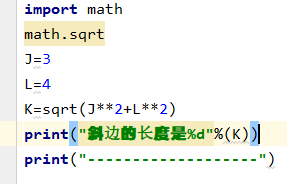3个回答

#coding=utf-8

``````import math
J = 3
L = 4
K = math.sqrt(J ** 2 + L ** 2)
print("斜边的长度是 %d"%(K))
print("---------------")
``````

## 斜边的长度是 5